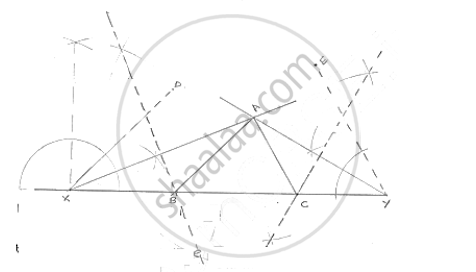# Using Ruler and Compasses Only, Construct a δAbc from the Following Data: Ab + Bc + Ca = 12 Cm, ∠B = 45° and ∠C = 60°. - Mathematics

Using ruler and compasses only, construct a ΔABC from the following data:
AB + BC + CA = 12 cm, ∠B = 45° and ∠C = 60°.

#### SolutionSteps of construction:
Step 1: Draw a line segment XY of 12cm.
Step 2: Draw ∠DXY = ∠B = 45° and ∠EYX = ∠C = 60°
Step 3: Draw the angle bisectors of angles of DXY and EYX which intersects each other at A.
Step 4: Draw the perpendicular of AX and AY which intersect XY at B and C respectively.
Step 5: Join AB and AC
∴Δ ABC is the required triangle

Concept: Some Constructions of Triangles
Is there an error in this question or solution?

#### APPEARS IN

RD Sharma Mathematics for Class 9
Chapter 16 Constructions
Exercise 16.3 | Q 9 | Page 18

Share